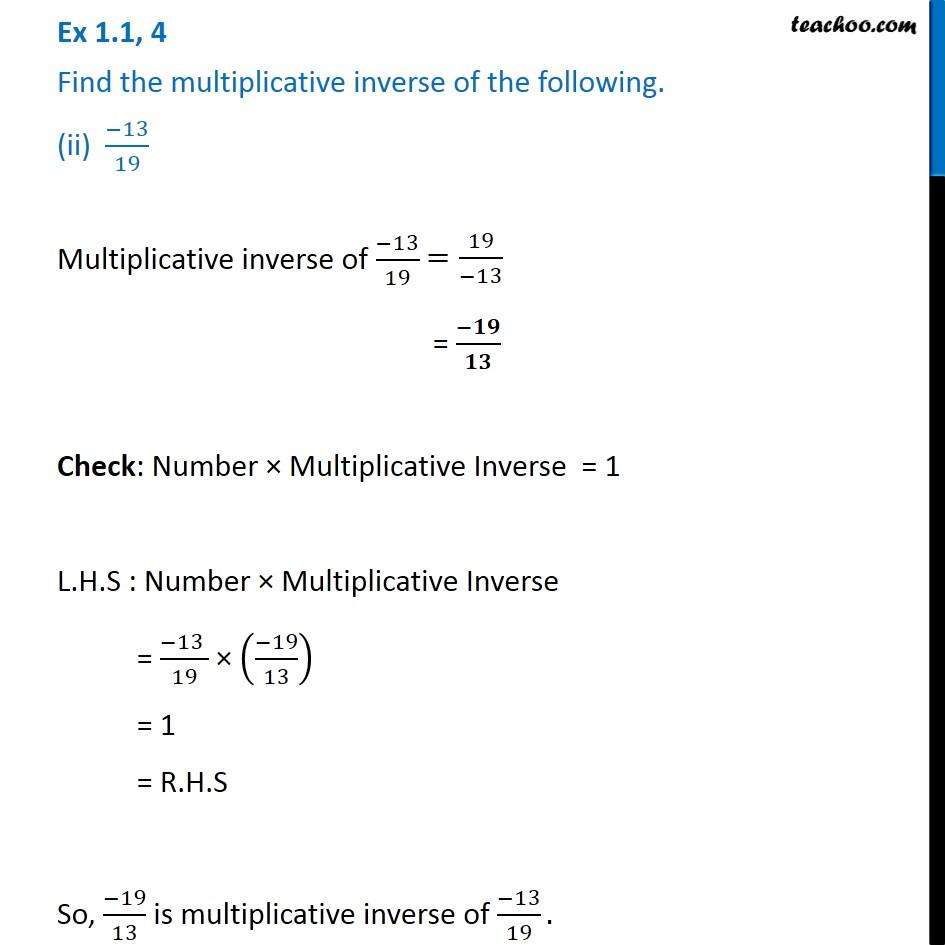Ex 1.1

Chapter 1 Class 8 Rational Numbers
Serial order wiseGet live Maths 1-on-1 Classs - Class 6 to 12

### Transcript

Ex 1.1, 4 Find the multiplicative inverse of the following. (ii) ( 13)/19 Multiplicative inverse of ( 13)/19 = ( )/ Check: Number Multiplicative Inverse = 1 L.H.S : Number Multiplicative Inverse = ( 13 )/19 (( 19)/13) = 1 = R.H.S So, ( 19)/13 is multiplicative inverse of ( 13)/19.